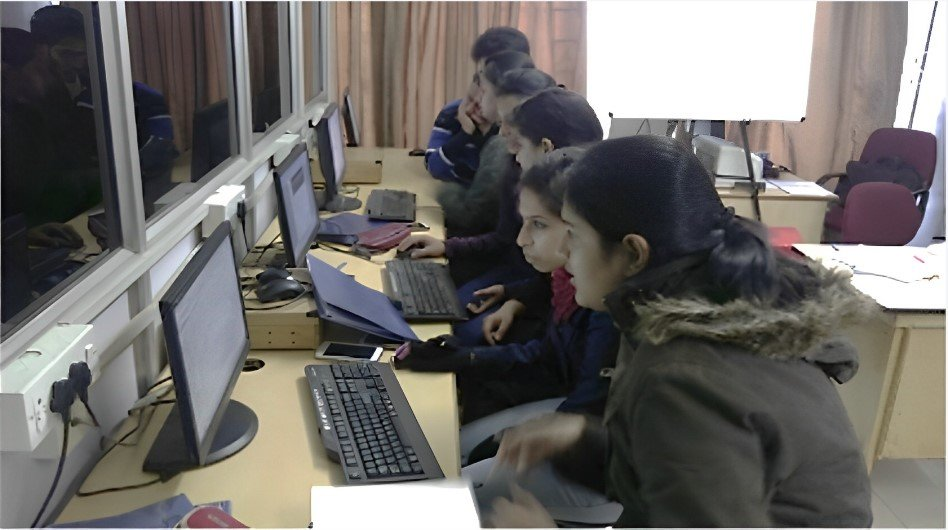1. Lab Room No: A308
2. Lab In charge: Dr. K. Sudha
3. Lab Attendant: Mr. Prem Singh
4. Lab Subjects: ADVANCED CONTROL SYSTEM – ETEE 453(VII SEM)About Lab:

This laboratory course covers the design and applications of advanced control systems. The aim of the course is to develop student knowledge and understanding of advanced control system design, simulation and implementation. It also aims to help the students apply their mathematical knowledge of control system theory to a number of practical examples. At the end of this course, students will be able to model and determine a suitable controller for the system, design a selected controller for the system, simulate to check the performance of the designed controller. Matlab/Simulink based exercises covering rigorous examples of modeling, design, simulation and implementation of control systems are included in this course. This laboratory aims to impart knowledge of state space, discrete systems, non-linear systems and adaptive control.

Hardware Details:  10 Computer (I-5 processor, RAM-8 GB,1 Printer)

Software Details: MATLAB 2017b 10 users

List of Experiments:

1. Study of open loop and closed loop time/ frequency responses of first/second order LTI system
2. Conversion of transfer functions to state model of LTI system and vice versa
3. Determine State Space Model of a given system and determine its controllability and observability.
4. Analysis of Zero order hold and first order hold circuits.
5. Conversion of transfer functions to state model of discrete time system.
6. To determine state transition matrix of a given system.
7. Study of saturation and dead zone non-linearity using describing function technique of a relay control system.
8. To draw phase trajectory of a given non-linear system.
9. Experiments based on PLC applications e.g., Lift control models, pick and place module etc.
10. Study of operation of a stepper motor interface with microprocessor.